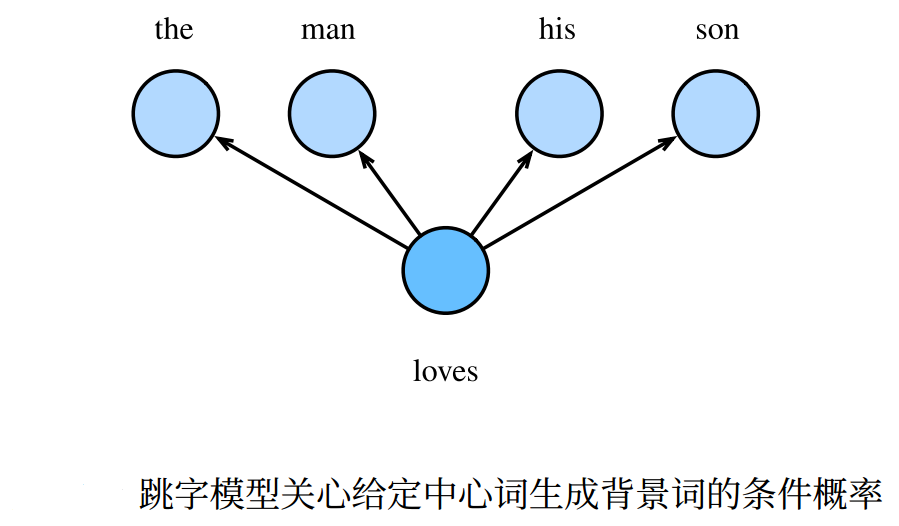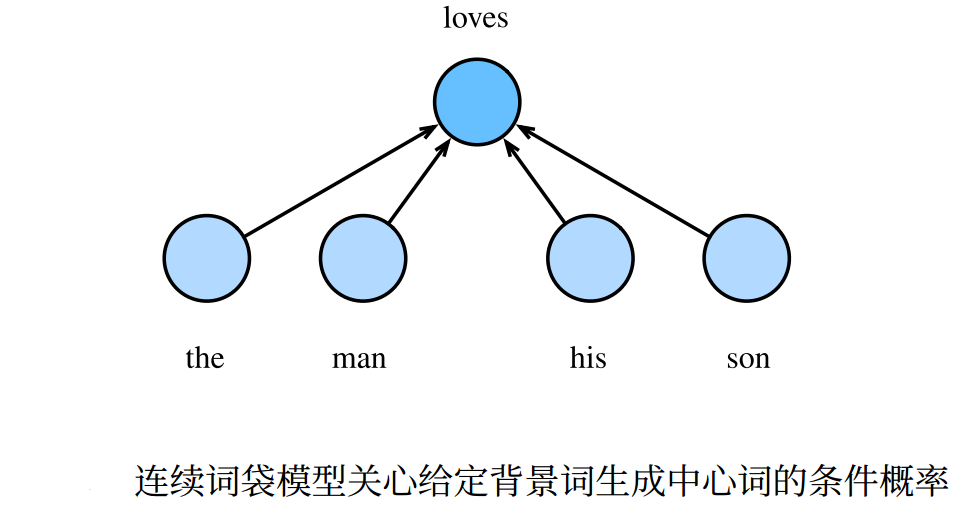# 词嵌入

【注】参考自李沐的《动手学深度学习》。

## 2. 词嵌入

• 最初的词嵌入采用 one-hot 编码来生成词向量，但 one-hot 编码的词向量无法准确表达不同词之间的相似度。刻画词向量间的相似度常常使用余弦相似度：对于向量 $\boldsymbol{x},\boldsymbol{y} \in \mathbb{R}^d$，它们之间的余弦相似度为

$\begin{array}{c} \frac{\boldsymbol{x}^T \boldsymbol{y}}{\parallel\boldsymbol{x}\parallel \parallel\boldsymbol{y}\parallel} \in [-1, 1] \end{array}$

• 目前的词嵌入采用 word2vec 工具，其主要包含两中词向量编码模型：跳字模型连续词袋模型

### 2.1 跳字模型

\begin{array}{c} P(\mbox{「the」},\mbox{「man」},\mbox{「his」},\mbox{「son」}|\mbox{「loves」}) = P(\mbox{「the」}|\mbox{「loves」}) \cdot P(\mbox{「man」}|\mbox{「loves」}) \cdot P(\mbox{「his」}|\mbox{「loves」}) \cdot P(\mbox{「son」}|\mbox{「loves」}) \end{array}• 在跳字模型中，每个词被表示为两个 $d$ 维向量，用来计算条件概率。假设某个词在词典中的索引为 $i$，则它作为中心词时词向量表示为 $\boldsymbol{v}_i \in \mathbb{R}^d$，作为背景词时词向量表示为 $\boldsymbol{u}_i \in \mathbb{R}^d$

• 设中心词 $w_c$ 在词典中索引为 $c$，背景词 $W_o$ 在词典中索引为 $o$，则给定中心词生成背景词的条件概率为

$\begin{array}{c} P(w_o | w_c) = \frac{e^{\boldsymbol{u}_o^T \boldsymbol{v}_c}}{\sum_{i \in \mathcal{V}} e^{\boldsymbol{u}_i^T \boldsymbol{v}_c}} \end{array}$

• 假设给定一个长度为 $T$ 的文本序列，设时间步 $t$ 的词为 $w^{(t)}$，当窗口大小为 $m$ 时，跳字模型的目标似然函数即为给定任一中心词生成其所有背景词的概率

$\begin{array}{c} \prod_{t=1}^T \prod_{-m \leq j \leq m, j \ne 0} P(w^{(t+j)} | w^{(t)}) \end{array}$

$\begin{array}{c} \ - \sum_{t=1}^T \sum_{-m \leq j \leq m, j \ne 0} \log P(w^{(t+j)} | w^{(t)}) \end{array}$

【注】在跳字模型中，一般使用中心词向量作为词向量表达词。

### 2.2 连续词袋模型

\begin{array}{c} P(\mbox{「loves」}|\mbox{「the」}, \mbox{「man」}, \mbox{「his」}, \mbox{「son」}) \end{array}• 在连续词袋模型中，每个词也被表示为两个 $d$ 维向量，用来计算条件概率。假设某个词在词典中的索引为 $i$，与跳字模型相反的是，则它作为背景词时词向量表示为 $\boldsymbol{v}_i \in \mathbb{R}^d$，作为中心词时词向量表示为 $\boldsymbol{u}_i \in \mathbb{R}^d$

• 由于背景词有多个，一般将这些背景词向量取平均后来计算条件概率。设中心词 $w_c$ 在词典中索引为 $c$，背景词 $w_{o_1},\cdots,w_{o_{2m}}$ 在词典中索引为 $o_1,\cdots,o_{2m}$，则给定背景词生成中心词的条件概率为：

$\begin{array}{c} P(w_c | w_{o_1}, \cdots, w_{o_{2m}}) = \frac{e^{\frac{1}{2m} \boldsymbol{u}_c^T (\boldsymbol{v_{o_1}} + \cdots + \boldsymbol{v_{o_{2m}}})}}{\sum_{i \in \mathcal{V}} e^{\frac{1}{2m} \boldsymbol{u}_i^T (\boldsymbol{v_{o_1}} + \cdots + \boldsymbol{v_{o_{2m}}})}} \end{array}$

$\mathcal{W}_o = \{w_{o_1}, \cdots, w_{o_{2m}}\}, \bar{\boldsymbol{v}}_o = (\boldsymbol{v_{o_1}} + \cdots + \boldsymbol{v_{o_{2m}}})$，则上式可简写成

$\begin{array}{c} P(w_c | \mathcal{W}_o) = \frac{e^{\boldsymbol{u}_c^T \bar{\boldsymbol{v}}_o}}{\sum_{i \in \mathcal{V}} e^{\boldsymbol{u}_i^T \bar{\boldsymbol{v}_o}}} \end{array}$

• 假设给定一个长度为 $T$ 的文本序列，设时间步 $t$ 的词为 $w^{(t)}$，当窗口大小为 $m$ 时，连续词袋模型的似然函数是由背景词生成任意中心词的概率

$\begin{array}{c} \prod_{t=1}^T P(w^{(t)} | w^{(t-m)}, \cdots, w^{(t-1)}, w^{(t+1)}, \cdots, w^{(t+m)}) \end{array}$

$\begin{array}{c} \ - \sum_{t=1}^T \log P(w^{(t)} | w^{(t-m)}, \cdots, w^{(t-1)}, w^{(t+1)}, \cdots, w^{(t+m)}) \end{array}$

【注】在连续词袋模型中，一般使用背景词向量作为词向量表达词。